+
Terms and Factors in Algebraic Expressions
Solving Linear Equations and Inequalities
0
of 0 possible points

# Terms and Factors in Algebraic Expressions

Author: Sophia Tutorial
##### Description:

Simplify an algebraic expression by combining like-terms.

(more)

Sophia’s self-paced online courses are a great way to save time and money as you earn credits eligible for transfer to many different colleges and universities.*

No credit card required

37 Sophia partners guarantee credit transfer.

299 Institutions have accepted or given pre-approval for credit transfer.

* The American Council on Education's College Credit Recommendation Service (ACE Credit®) has evaluated and recommended college credit for 33 of Sophia’s online courses. Many different colleges and universities consider ACE CREDIT recommendations in determining the applicability to their course and degree programs.

Tutorial
what's covered
1. Terms in Algebraic Expressions
2. Types of Algebraic Expressions
3. Parts of an Algebraic Expression
4. Combining Like Terms

# 1. Terms and Factors in Algebraic Expressions

The following is a single term: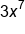Here we see the two separate quantities 3 and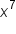multiplied by one another. We can also dissect the expression a little further we get 3•x•x•x•x•x•x•x. Notice how all numbers and variables are combined through multiplication only. We say that the above example represents a single term.

term to know
Term
A collection of numbers, variables, and powers combined through multiplication.

# 2. Types of Algebraic Expressions

When dealing with algebraic expressions the number of different terms that are added to or subtracted from one another give the expression a different name. Here we will look at different types of expressions based on the number of unique terms they contain.

The simplest algebraic expression is just a number, such as 3. A single number is called a constant, or a term that is not multiplied by a variable.

A single algebraic expressions with no other terms added to or being subtracted from it is called a monomial. For example,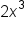is a monomial.

hint
A constant is a special type of monomial where there are no variables being multiplied to a number. For example, 5 or 14 are constants.

Typically when writing algebraic expressions we refer to them using their variable and the power the variable is being raised to. For example,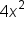would be called a second degree monomial because it the variable is being raised to the second power.

More complex types of algebraic expression contains more than one monomial and are combined through either addition or subtraction.

If we have two monomials combined with one another we call that expression a binomial. For example,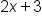and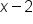are binomials.

If we have more than two monomials combined with one another we have what is called a polynomial. For example,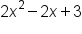is a polynomial. We typically say that this expression is a second degree polynomial because the highest power of any variable in the expression is 2. If we had the expression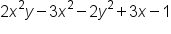, we would count the total number of powers in each monomial to determine the power. In this example we would say that this is a 3rd degree polynomial because in the term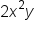the combined power of x and y add up to 3.

# 3. Parts of an Algebraic Expression

When working with algebraic expressions you should be familiar with the parts that make up the expression. Here we will discuss and identify coefficients, variables, powers or degree, and constant terms.

If we have the expression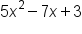, the variable represents an unknown quantity, and is typically written as a letter. In this case the variable would be x. Coefficients would be the number in front of a variables. In this case the 5 and 7 would be coefficients. The power or degree of this polynomial is 2 since that is the highest power variables are being raised to. Finally, the constant would be 3 since that is the only term without a variable component.

Now let’s look at how to combine two or more algebraic expressions.

# 4. Combining Like-Terms

One way we can simplify expressions is to combine like-terms. Like-terms are terms where the variables match exactly (exponents included). Examples of like-terms would be 3xy and −7xy or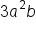and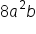or −3 and 5. If we have like-terms we are allowed to add (or subtract) the numbers in front of the variables, then keep the variables the same. This is shown in the following examples.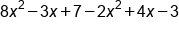Combine like terms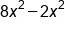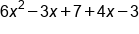Combine like terms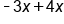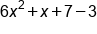Combine like terms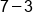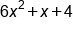Our Solution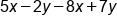Combine like terms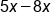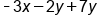Combine like terms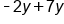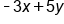Our Solution

As we combine like-terms, we need to interpret subtraction signs as part of the following term. This means if we see a subtraction sign, we treat the following term like a negative term, the sign always stays with the term.

summary
When considering terms and factors in algebraic expressions, we can define term as a collection of numbers, variables, and powers. Types of algebraic expressions can be monomials, binomials, and polynomials. Parts of an algebraic expression include the variables, corresponding coefficients, powers, and constants. Terms are referred to by their variable and their power or exponent. When combining like-terms, we are combining terms that have the same variable and the same power with addition and subtraction.

Source: Adapted from "Beginning and Intermediate Algebra" by Tyler Wallace, an open source textbook available at: http://wallace.ccfaculty.org/book/book.html

Terms to Know
Algebraic Expression

A combination of numbers, variables, and operators representing a quantity.

Coefficient

The number in front of a variable term that acts as a factor or multiplier.

Constant

A term with no variable component.

Factor

A number or quantity used in multiplication.

Polynomial

An expression containing several terms.

Term

A collection of numbers, variables, and powers combined through multiplication.

Variable

A quantity that can change, expressed as a letter or symbol.

Rating